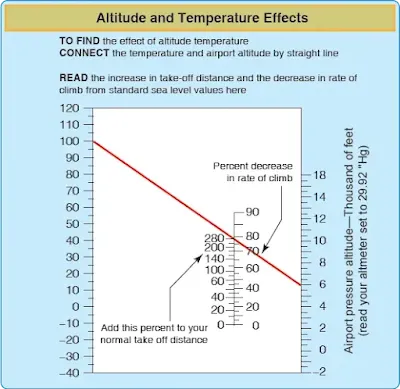# Atmosphere

The atmosphere is the envelope of air which surrounds the Earth. A given volume of dry air contains about 78 percent nitrogen, 21 percent oxygen, and about 1 percent other gases such as argon, carbon dioxide, and others to a lesser degree.

Although seemingly light, air does have weight and a one square inch column of the atmosphere at sea level weighs approximately 14.7 pounds. About one-half of the air by weight is within the first 18,000 feet. The remainder of the air is spread over a vertical distance in excess of 1,000 miles.

Air density is a result of the relationship between temperature and pressure. Air density is inversely related to temperature and directly related to pressure. For a constant pressure to be maintained as temperature increases, density must decrease, and vice versa. For a constant temperature to be maintained as pressure increases, density must increase, and vice versa. These relationships provide a basis for understanding instrument indications and aircraft performance.

## Layers of the Atmosphere

There are several layers to the atmosphere with the troposphere being closest to the Earth’s surface extending to about 60,000 feet at the equator. Following is the stratosphere, mesosphere, ionosphere, thermosphere, and finally the exosphere. The tropopause is the thin layer between the troposphere and the stratosphere. It varies in both thickness and altitude but is generally defined where the standard lapse (generally accepted at 2 °C per 1,000 feet) decreases significantly (usually down to 1 °C or less).

## International Standard Atmosphere (ISA)

The International Civil Aviation Organization (ICAO) established the ICAO Standard Atmosphere as a way of creating an international standard for reference and performance computations. Instrument indications and aircraft performance specifications are derived using this standard as a reference. Because the standard atmosphere is a derived set of conditions that rarely exist in reality, pilots need to understand how deviations from the standard affect both instrument indications and aircraft performance.

In the standard atmosphere, sea level pressure is 29.92 inches of mercury ("Hg) and the temperature is 15 °C (59 °F). The standard lapse rate for pressure is approximately a 1 "Hg decrease per 1,000 feet increase in altitude. The standard lapse rate for temperature is a 2 °C (3.6 °F) decrease per 1,000 feet increase, up to the top of the stratosphere. Since all aircraft performance is compared and evaluated in the environment of the standard atmosphere, all aircraft performance instrumentation is calibrated for the standard atmosphere. Because the actual operating conditions rarely, if ever, fit the standard atmosphere, certain corrections must apply to the instrumentation and aircraft performance. For instance, at 10,000 ISA predicts that the air pressure should be 19.92 "Hg (29.92 "Hg – 10 "Hg = 19.92 "Hg) and the outside temperature at –5 °C (15 °C – 20 °C). If the temperature or the pressure is different than the International Standard Atmosphere (ISA) prediction an adjustment must be made to performance predictions and various instrument indications.

### Pressure Altitude

Pressure altitude is the height above the standard datum plane (SDP). The aircraft altimeter is essentially a sensitive barometer calibrated to indicate altitude in the standard atmosphere. If the altimeter is set for 29.92 "Hg SDP, the altitude indicated is the pressure altitude-the altitude in the standard atmosphere corresponding to the sensed pressure.

The SDP is a theoretical level where the pressure of the atmosphere is 29.92 "Hg and the weight of air is 14.7 psi. As atmospheric pressure changes, the SDP may be below, at, or above sea level. Pressure altitude is important as a basis for determining aircraft performance, as well as for assigning flight levels to aircraft operating at or above 18,000 feet. The pressure altitude can be determined by either of two methods: (1) by setting the barometric scale of the altimeter to 29.92 "Hg and reading the indicated altitude, or (2) by applying a correction factor to the indicated altitude according to the reported altimeter setting.

### Density Altitude

Density altitude is pressure altitude corrected for nonstandard temperature. As the density of the air increases (lower density altitude), aircraft performance increases. Conversely, as air density decreases (higher density altitude), aircraft performance decreases. A decrease in air density means a high density altitude; an increase in air density means a lower density altitude. Density altitude is used in calculating aircraft performance. Under standard atmospheric conditions, air at each level in the atmosphere has a specific density; under standard conditions, pressure altitude and density altitude identify the same level. Density altitude, then, is the vertical distance above sea level in the standard atmosphere at which a given density is to be found. It can be computed using a Koch Chart or a flight computer with a density altitude function. [Figure]Koch chart sample

If a chart is not available, the density altitude can be estimated by adding 120 feet for every degree Celsius above the ISA. For example, at 3,000 feet PA, the ISA prediction is 9 °C (15 °C – [lapse rate of 2 °C per 1,000 feet x 3 = 6 °C]). However, if the actual temperature is 20 °C (11 °C more than that predicted by ISA) then the difference of 11 °C is multiplied by 120 feet equaling 1,320. Adding this figure to the original 3,000 feet provides a density altitude of 4,320 feet (3,000 feet + 1,320 feet).

RELATED POSTS